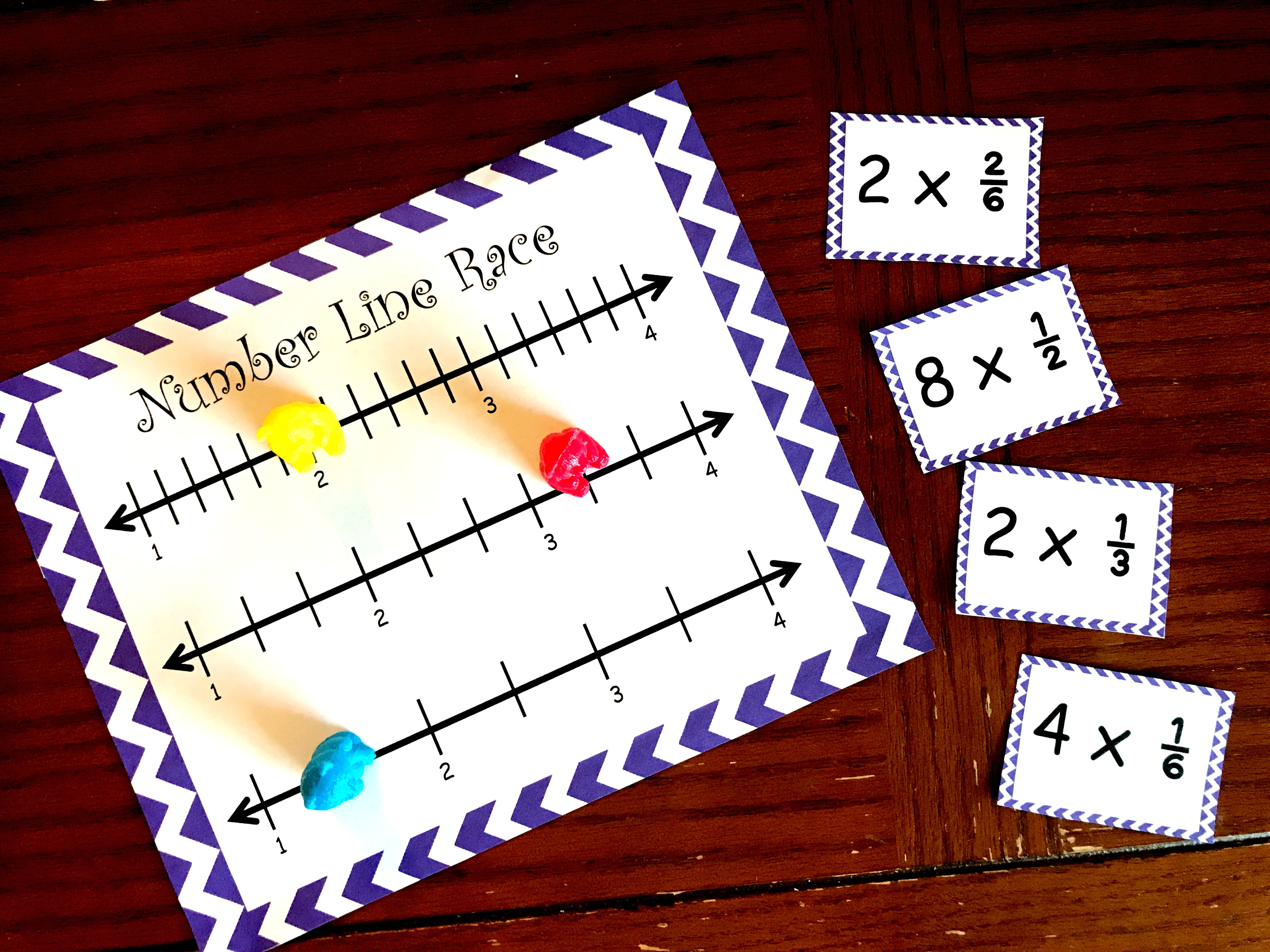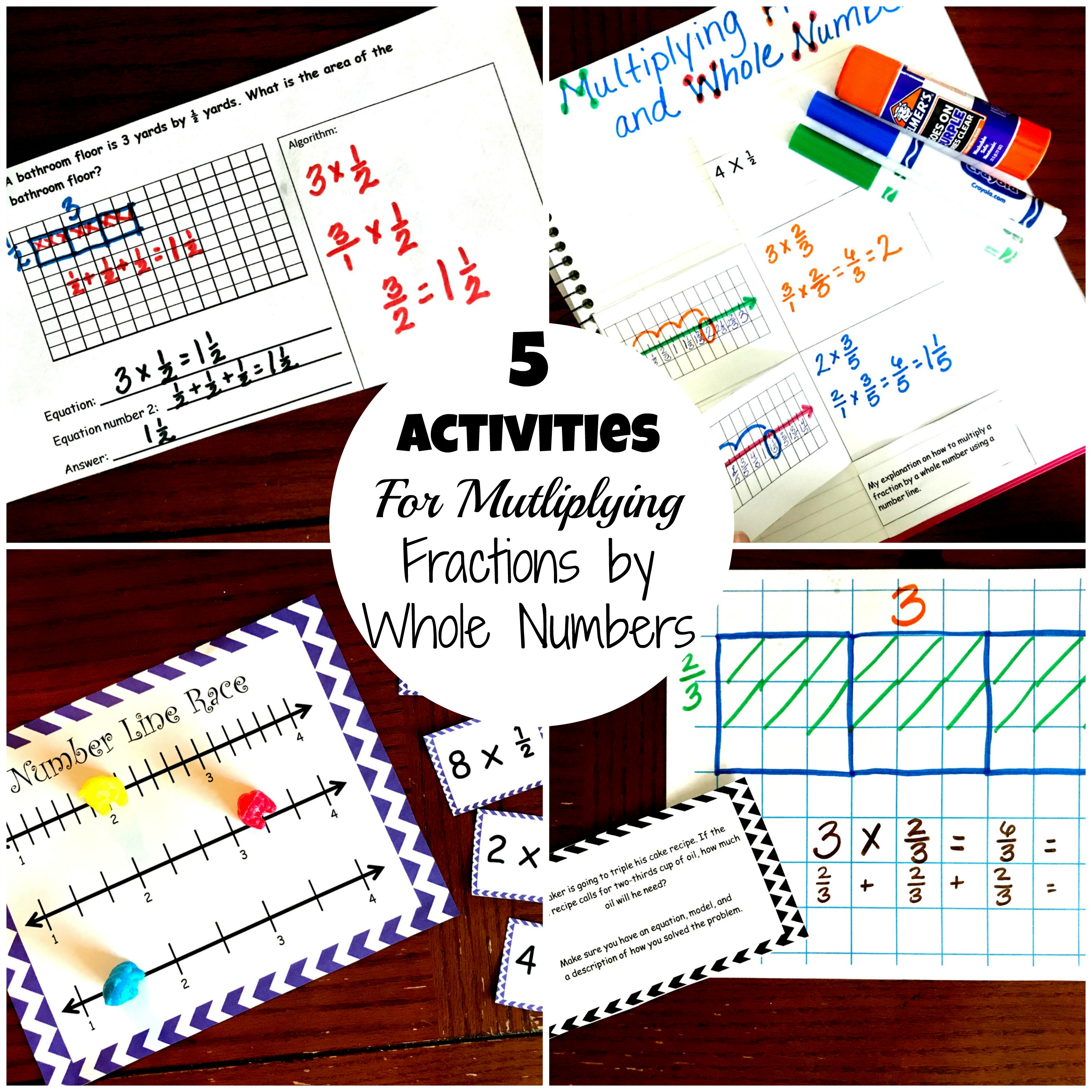Home » Math » 20 Word Problems for Multiplying Fractions By Whole Numbers

20 Word Problems for Multiplying Fractions By Whole Numbers

Grab these 20-word problems that get children multiplying fractions by whole numbers using real life examples. The cards also encourage children to model.The free printable can be found at the END of this post. Click on the blue “get your free printable here” button.

One of my favorite teaching positions in the public school was my time spent as a math coach. I thoroughly enjoyed having the time to really research the math we were learning and finding creative ways for us to teach them.

One goal we took on, was to keep a math journal. Three to five times a week the students would pull out their math journals and solve word problems in them. Our upper elementary students had grids at the top and lined paper at the bottom. We spent a lot of time teaching them how to use the grids to correctly model their work and spent just as much time modeling a good explanation for how they solved the problems.

Trust me when I say that neither of those is easy to teach. There is a lot of planning that must go into a correctly drawn model or number line. For example, if I’m going to draw a number line to show equivalent fractions for fourths and eighths, my number line had better be at least eight squares long for both number lines. We all know how writing explanations go. Any writing, whether fiction of non-fiction, needs to be modeled numerous times and writing for math is no exception.

My fifth-grade teachers really embraced this, and we saw tremendous growth in our students. We weren’t doing it for test scores, but it was fun to see some awesome math scores when they came back in May.

So what did we do?

First, every child was provided with a notebook that had grid paper in it. Then we created word problems that went with our unit. At the beginning of the unit, we often started off with easy one-step problems but quickly moved into multi-step problems. Our team also did not always have problems that included our topic. We didn’t want the children to get into the thought process that since we were studying multiplying fraction that they would need to multiply to solve the problem.

What should each answer have?

Every problem they solved was required to have four things.

1. First, they needed an answer.
2. Next, they also had to include a model.
3. An equation also had to be included.
4.  Finally, they had to explain in words how they solved their problem.

Multiply Fractions by Whole Numbers Word Problems

This problem gives the dimensions of two different pieces of land. The students have to figure out who has the largest piece and how much more they have. In this problem alone the children are multiplying whole numbers by fractions twice, determining which fraction is greater, and then subtracting fractions.

If you look at the models in this picture, you can see the pre-planning that went into it. One whole equals four squares for both models. This gives the student a solid line for one-half and makes it easy to draw their own line for 3/4. Also note how each box has the fraction of it, and then an equation at the bottom adding up all the fractions in the box. This is just another way for the students to show that they know what is happening when they multiply whole numbers.For this example, the student actually has three equations. They have an equation for each model and then the equation at the end allows them to solve the whole problem using that one equation. That step really requires students to understand the process they used to solve. You can’t just haphazardly write down some numbers and the equation work!!!!

Getting in these 20 Word Problems or other Word Problems

And how do you work these into your math time??? Well if you homeschool, that is simple. I would recommend doing it before you start math each day. Public or private school? There are many different ideas. Some teachers have them as morning work, and they need to be completed before the day starts. Others have done them as part of a center. The students work on them while you are meeting with a small group. Some just start their math class with 10 minutes of everyone sitting down and solving a problem.

The hardest part though is making sure you go over every problem thoroughly. How can it be modeled? What equation did you come up with? Read your explanation!! This is where the learning comes in, and where you are going to help your students improve their answers and problem-solving skills.

Good luck on working with your kiddos on these fun word problems.

You’ve Got This

You may also like:

THis Number line GameOr get all my multiplying fractions by whole numbers in one place.